# SOLVE B AND C USING CASTIGLIANO'S THEOREM Consider the cantilever beam shown in . Suppose that...

SOLVE B AND C USING CASTIGLIANO'S THEOREM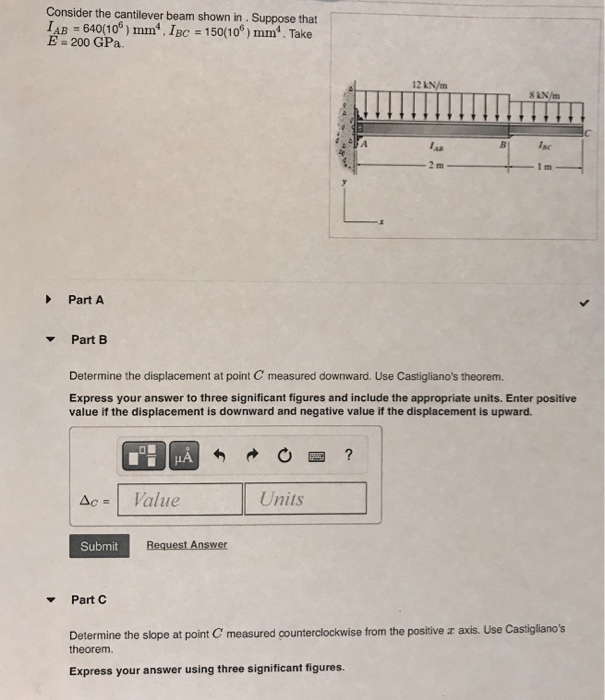Consider the cantilever beam shown in . Suppose that LAB = 640(106) mm IBC = 150(106) mm". Take E 200 GPa 12 kN/m 8 kN/m موا Inc Part A Part B Determine the displacement at point C measured downward. Use Castigliano's theorem. Express your answer to three significant figures and include the appropriate units. Enter positive value if the displacement is downward and negative value if the displacement is upward. HA ? Ac Value Units Submit Request Answer Part C Determine the slope at point C measured counterclockwise from the positive x axis. Use Castigliano's theorem Express your answer using three significant figures.

This Homework Help Question: "SOLVE B AND C USING CASTIGLIANO'S THEOREM Consider the cantilever beam shown in . Suppose that..." No answers yet.

We need 10 more requests to produce the answer to this homework help question. Share with your friends to get the answer faster!

0 /10 have requested the answer to this homework help question.

Once 10 people have made a request, the answer to this question will be available in 1-2 days.
All students who have requested the answer will be notified once they are available.

#### Earn Coin

Coins can be redeemed for fabulous gifts.

Similar Homework Help Questions
• ### Consider the cantilever beam shown in (Figure 1). Suppose that I AB = 800(106) mm4, IBC...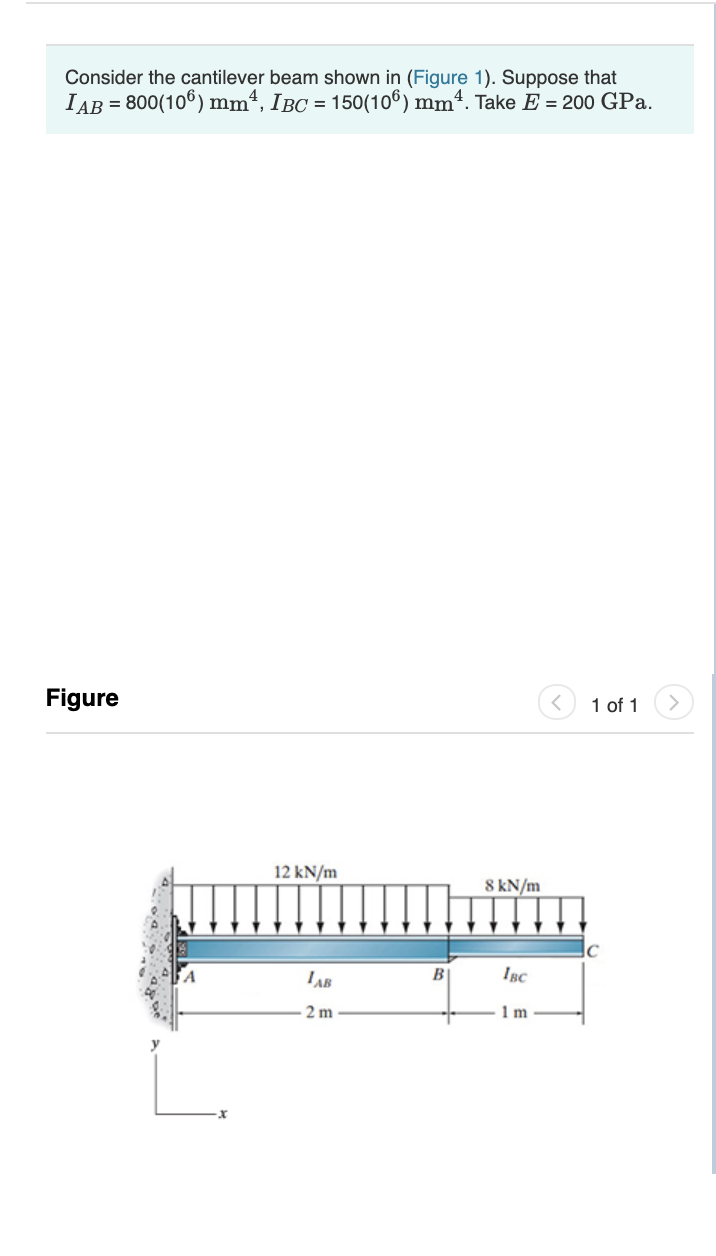Consider the cantilever beam shown in (Figure 1). Suppose that I AB = 800(106) mm4, IBC = 150(106) mm4. Take E = 200 GPa. Figure < 1 of 1 > 12 kN/m 8 kN/m 1AB 2 m B BC lm Part B Determine the displacement at point C measured downward. Use Castigliano's theorem. Express your answer to three significant figures and include the appropriate units. Enter positive value if the displacement is downward and negative value if the displacement is...

• ### Review Part B Consider the beam shown in (Figure 1). El is constant. Suppose that EI...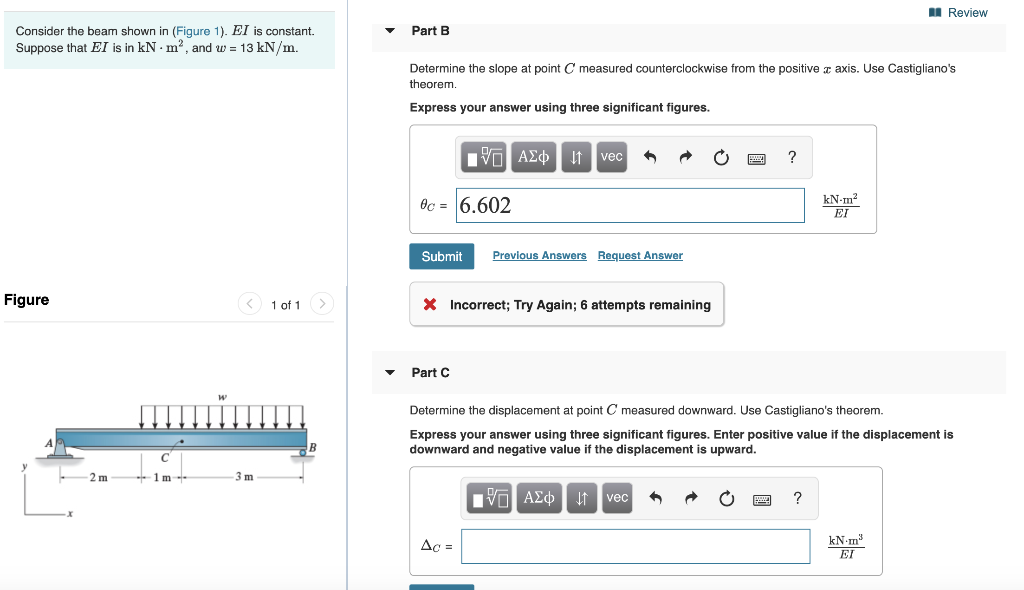Review Part B Consider the beam shown in (Figure 1). El is constant. Suppose that EI is in kNm", and w = 13 kN/m. Determine the slope at point C measured counterclockwise from the positive 3 axis. Use Castigliano's theorem. Express your answer using three significant figures. PO ASC u veca O ? 06 = 6.602 Submit Previous Answers Request Answer Figure < 1 of 1 X Incorrect; Try Again; 6 attempts remaining Part Determine the displacement at point C...

• ### Consider the beam shown in (Figure 1). Assume the support at A is a pin and...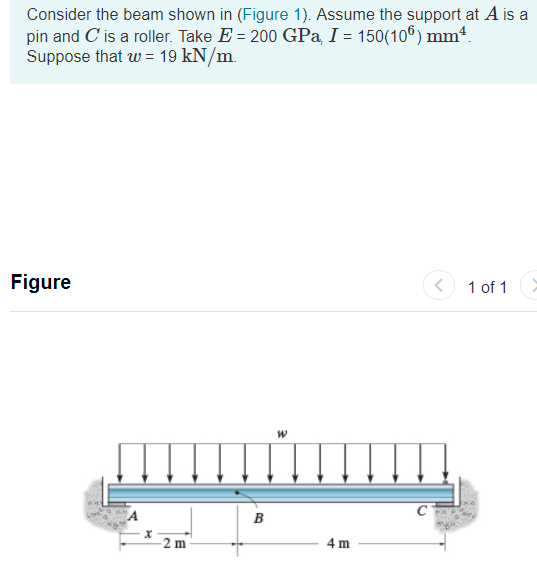Consider the beam shown in (Figure 1). Assume the support at A is a pin and C is a roller. Take E 200 GPa, I 150(106) mm Suppose that w 19 kN/m Figure 1 of 1 TA 2 m 4 m Part B Determine the slope at point B measured clockwise from the positive r axis. Use the method of virtual work Express your answer using three significant figures. rad Part Determine the displacement at point B measured downward. Use...

• ### HWK #16 Problem 9.26 3 of 3 Consider the cantilever beam shown in (Figure 1). Take...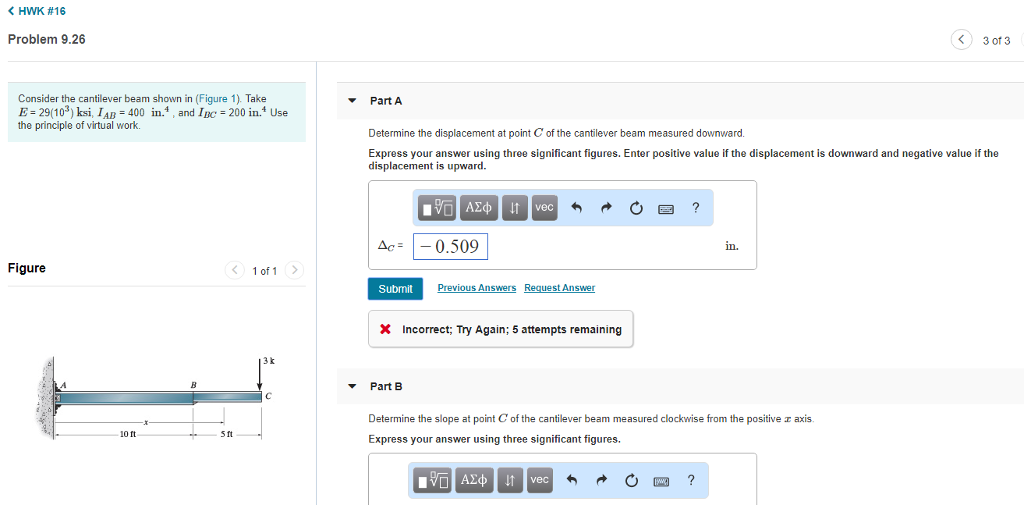HWK #16 Problem 9.26 3 of 3 Consider the cantilever beam shown in (Figure 1). Take E- 29(103) ksi, IAB 400 in., and IC 200 in.4 Use the principle of virtual work. Part A Determine the displacement at point C of the cantilever beam measured downward Express your answer using three significant figures. Enter positive value if the displacement is downward and negative value if the displacement is upward. Ac: 0.509 in. Figure < 1of1 > Submit vious Ans uest...

• ### Homework 11: moment area Problem 7.24 Part B Review Consider the beam shown in (Figure 1)....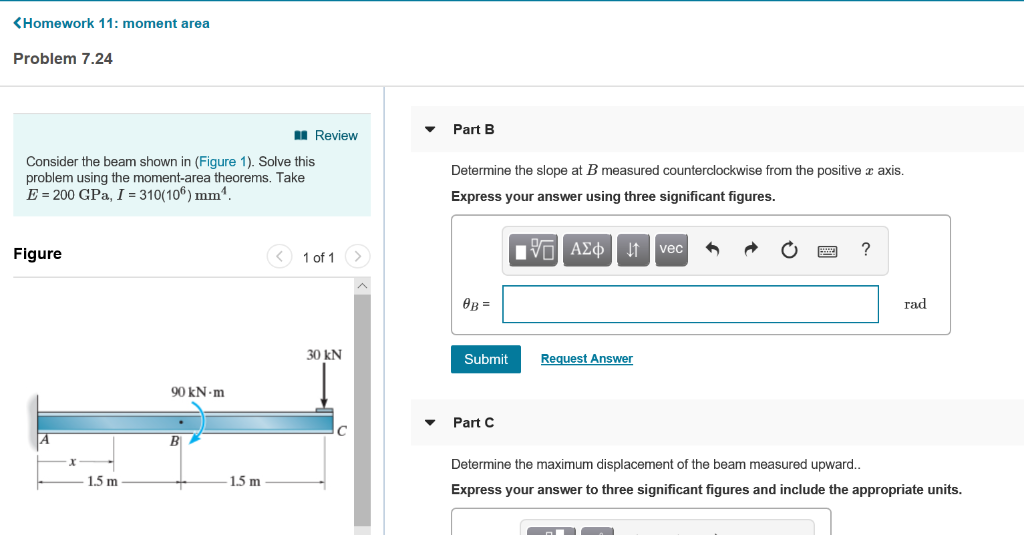Homework 11: moment area Problem 7.24 Part B Review Consider the beam shown in (Figure 1). Solve this problem using the moment-area theorems. Take E-200 GPa, 1-310(106) mm'. Determine the slope at B measured counterclockwise from the positive r axis Express your answer using three significant figures. vec Figure 1 of 1 rad 30 kN Submit Re st Answer 90 kN-m Part C Determine the maximum displacement of the beam measured upward Express your answer to three significant figures and...

• ### Consider the beam shown in (Figure 1). E = 200 GPa and I = 62(106) mm...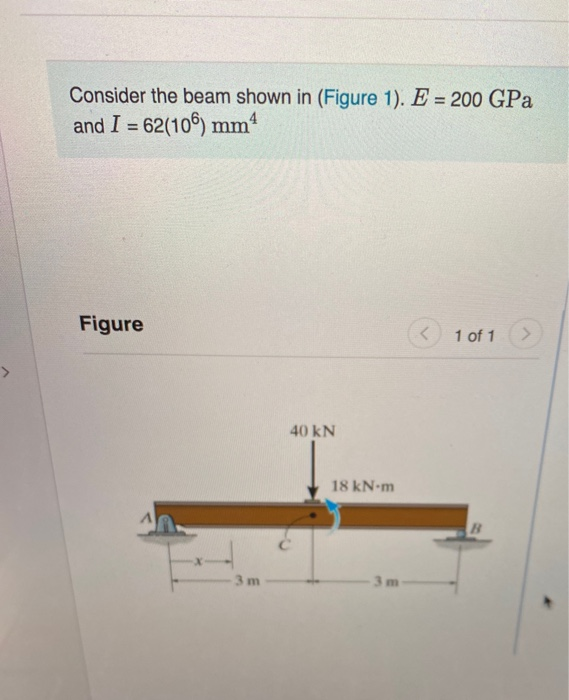Consider the beam shown in (Figure 1). E = 200 GPa and I = 62(106) mm 4 Figure 1 of 1 40 KN 18 kN.m Part A Determine the slope of the beam at B, measured counterclockwise from the positive x axis. Express your answer using three significant figures. V AED 11 vec t o Bera ? OB rad Submit Request Answer Part B Determine the deflection of the beam at C. Express your answer to three significant figures and...

• ### Part A A of the beam, measured counterclockwise from the positive in (Figure 1). E 200 GPa Determine the slope at axis....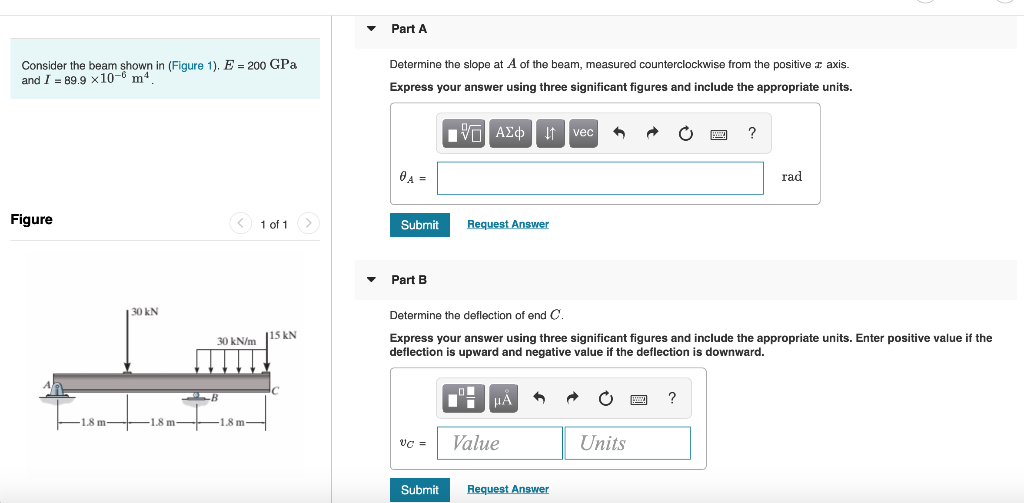Part A A of the beam, measured counterclockwise from the positive in (Figure 1). E 200 GPa Determine the slope at axis. Consider the b dI89 9 x10- m4 Express your answer using three significant figures and include the appropriate units. VA D vec rad Figure > Submit Request Answer 1 of 1 Part B 30 kN Determine the deflection of end C. 15 kN t ur answer using three sig the deflo clude the appropriate units. Enter positive value...

• ### Question 3 Consider the beam shown in (Figure 1). El is constant. Assume B and C...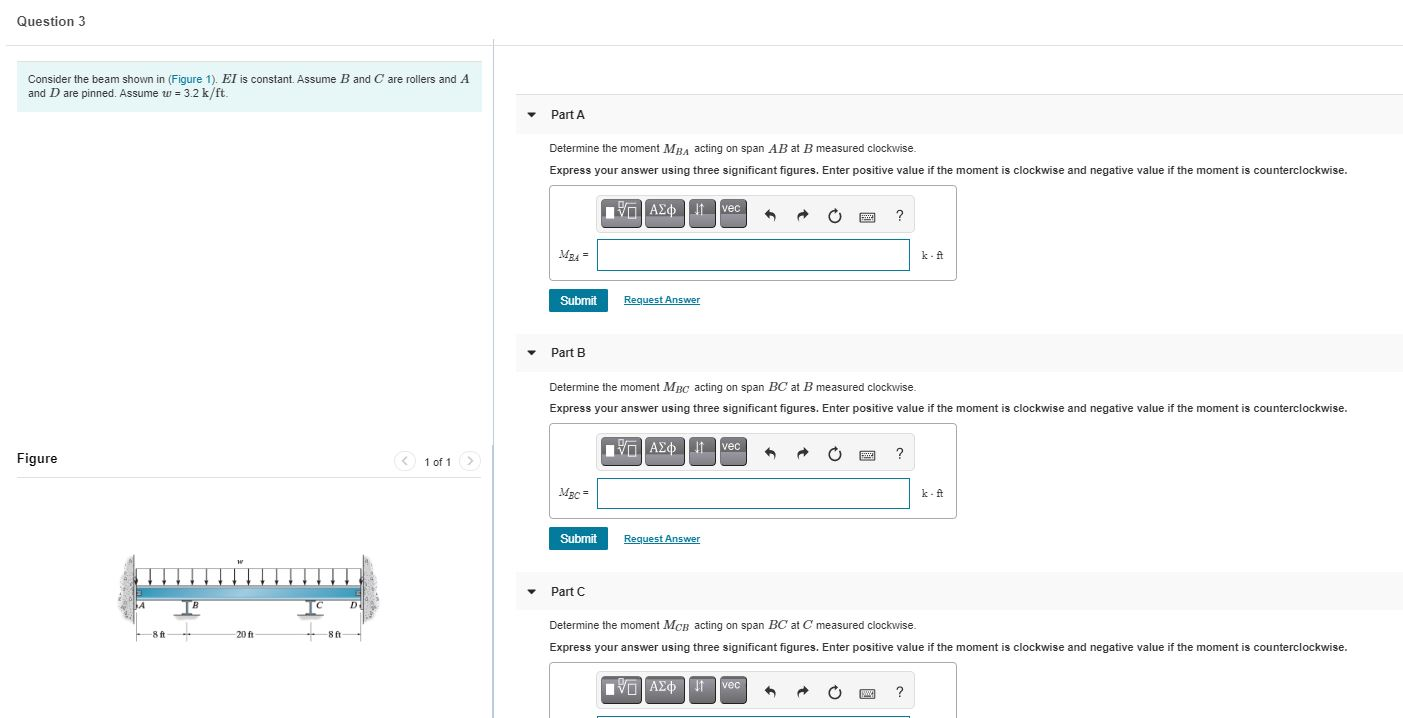Question 3 Consider the beam shown in (Figure 1). El is constant. Assume B and C are rollers and A and D are pinned. Assume u = 3.2 k/ft Part A Determine the moment MBA acting on span AB at B measured clockwise. Express your answer using three significant figures. Enter positive value if the moment is clockwise and negative value if the moment is counterclockwise. VOAP vec ? MBA Submit Request Answer Part B Determine the moment MBC acting...

• ### Question 3 Consider the beam shown in (Figure 1). El is constant. Assume B and C...Question 3 Consider the beam shown in (Figure 1). El is constant. Assume B and C are rollers and A and D are pinned. Assume u = 3.2 k/ft Part A Determine the moment MBA acting on span AB at B measured clockwise. Express your answer using three significant figures. Enter positive value if the moment is clockwise and negative value if the moment is counterclockwise. VOAP vec ? MBA Submit Request Answer Part B Determine the moment MBC acting...

• ### Question 3 Consider the beam shown in (Figure 1). El is constant. Assume B and C...Question 3 Consider the beam shown in (Figure 1). El is constant. Assume B and C are rollers and A and D are pinned. Assume u = 3.2 k/ft Part A Determine the moment MBA acting on span AB at B measured clockwise. Express your answer using three significant figures. Enter positive value if the moment is clockwise and negative value if the moment is counterclockwise. VOAP vec ? MBA Submit Request Answer Part B Determine the moment MBC acting...